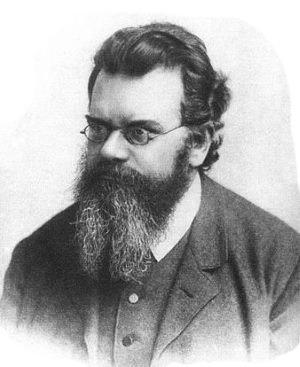calculator.org

# property>Boltzmann constant

## What is the Boltzmann Constant?Named after Austrian physicist Ludwig Boltzmann, the Boltzmann constant is considered to be a bridge between energy at the particle level and temperature at the bulk level. This relationship is one that moves from the macroscopic level to the microscopic level, using the ideal gas law. To get the Boltzmann constant mathematically, we simply divide R, the gas constant by Avogadro's number. In other words, the following equation is true:

k = R/NA

k, of course, stands for the Boltzmann constant. This calculation results in the number 1.3806504 x 10-23 in JK-1. We get the bridge between macroscopic and microscopic physics by substituting k*NA for R. Originally, the ideal gas equation is written as:

pV = nRT

where p is the pressure, V is the volume, n is the amount of substance, and T is the absolute temperature. The new equation with the Boltzmann constant substitution is written as:

V = NkT,

where N is the number of molecules of gas present (remember that NA*n will always yield the amount of molecules, or N). With this rather simple substitution, we find that the Boltzmann constant plays many different roles in thermodynamics.

For any kind of thermodynamic system at any kind of pre-defined temperature, each degree of freedom in this system, on the microscopic scale, has a magnitude of k*(T/2). We can apply this in simple gas thermodynamics when we consider monatomic ideal gasses, each of which always has three degrees of freedom (diatomic gases have an average of five, and other molecules can have many more), we find that each atom in a system will have an average thermal energy of 1.5kT. The experimental data supports this conclusion. Using this average thermal energy value, we can find the root mean square speed for monatomic gasses such as helium and xenon. We can then compare that calculation with observed values (1370 m/s for helium and 240 m/s for xenon). As it turns out, the calculation of root mean square speed using the Boltzmann equation and degrees of freedom to determine the total energy accurately predicts these speeds.

Engraved into Ludwig Boltzmann’s tombstone is the following mathematical formula:

S = k*log(W)

For Americans, this is taken to mean S = k*ln(W) because we assume that "log" means a base-10 logarithm.

This equation is considered central to statistical mechanics, for it defines the entropy of an isolated system at thermodynamic equilibrium. W stands for the number of distinct microscopic states in the system based on certain macroscopic constraints such as energy and temperature. Ultimately, this equation defines the relationship between a system’s microscopic and macroscopic states because all microscopic states are represented through W and all macroscopic states are represented with S. The Boltzmann constant, once again, serves as the bridge between these two levels.

The Boltzmann constant is found in semiconductor physics as well. Wherever there is a p-n junction, a voltage characteristic called thermal voltage (VT) can be related to the absolute temperature of the system with this equation:

VT = (kT)/q

Here, q is taken to be the magnitude to the electrical charge on the electron, a constant equal to 1.602 x 10-19. At room temperature, it is fairly easy to calculate this number, which approximately 25.85 millivolts.

Bookmark this page in your browser using Ctrl and d or using one of these services: (opens in new window)